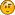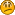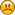# On the subject of a curve

• 21 Replies
• 1894 Views#### Scroto Gaggins

• 671
• Hobbiton represent##### On the subject of a curve
« on: March 19, 2015, 01:37:43 AM »
Lots of FE'rs, trolls or not, cite the common 'proof' of the earths flatness as the fact that one cannot see curvature on the ground. People have then tried to explain that the earth is too big to notice any difference. People like JRowe have been very adamant that a curved line cannot look flat.
They're wrong. Reasons why:
Consider a Cartesian plane. On it, an exponential graph of the formula y=2x
I cant post one, but a quick google search will show what i mean.
It is quite clearly a curve, any asshole can see that.
But if one looks at the 'tail' end of the line (i.e. x<-2 or so) then it begins to look rather flat.
When you look at the coordinates, it is most assuredly a curve.
Similar principal with the curvature on the horizon.

tl;dr- the horizon can be curved without it being noticeable. Maths
They are taking the hobbits to Isengard.

?

#### herewegoround

• 286##### Re: On the subject of a curve
« Reply #1 on: March 19, 2015, 03:58:06 AM »
Lots of FE'rs, trolls or not, cite the common 'proof' of the earths flatness as the fact that one cannot see curvature on the ground. People have then tried to explain that the earth is too big to notice any difference. People like JRowe have been very adamant that a curved line cannot look flat.
They're wrong. Reasons why:
Consider a Cartesian plane. On it, an exponential graph of the formula y=2x
I cant post one, but a quick google search will show what i mean.
It is quite clearly a curve, any asshole can see that.
But if one looks at the 'tail' end of the line (i.e. x<-2 or so) then it begins to look rather flat.
When you look at the coordinates, it is most assuredly a curve.
Similar principal with the curvature on the horizon.

tl;dr- the horizon can be curved without it being noticeable. Maths

Curves lines are locally straight, curved surfaces are locally flat; both approximately. It's a well understood concept in mathematics. It can be tested easily. Draw a large circle a few metres in diameter on the ground. Mark off two point on the circle about 1 cm apart. Rub out the rest of the circle and the bit left will look straight.#### FalseProphet

• 3696
• Life is just a tale##### Re: On the subject of a curve
« Reply #2 on: March 19, 2015, 04:28:39 AM »
If curved lines can look straight, why can straight lines never look curved?#### Scroto Gaggins

• 671
• Hobbiton represent##### Re: On the subject of a curve
« Reply #3 on: March 19, 2015, 04:46:59 AM »
Lots of FE'rs, trolls or not, cite the common 'proof' of the earths flatness as the fact that one cannot see curvature on the ground. People have then tried to explain that the earth is too big to notice any difference. People like JRowe have been very adamant that a curved line cannot look flat.
They're wrong. Reasons why:
Consider a Cartesian plane. On it, an exponential graph of the formula y=2x
I cant post one, but a quick google search will show what i mean.
It is quite clearly a curve, any asshole can see that.
But if one looks at the 'tail' end of the line (i.e. x<-2 or so) then it begins to look rather flat.
When you look at the coordinates, it is most assuredly a curve.
Similar principal with the curvature on the horizon.

tl;dr- the horizon can be curved without it being noticeable. Maths

Curves lines are locally straight, curved surfaces are locally flat; both approximately. It's a well understood concept in mathematics. It can be tested easily. Draw a large circle a few metres in diameter on the ground. Mark off two point on the circle about 1 cm apart. Rub out the rest of the circle and the bit left will look straight.

I know, thats why i posted
They are taking the hobbits to Isengard.#### Scroto Gaggins

• 671
• Hobbiton represent##### Re: On the subject of a curve
« Reply #4 on: March 19, 2015, 04:49:59 AM »
If curved lines can look straight, why can straight lines never look curved?Because a straight line by definition, cant be curved.
Get it right, Noriega!
They are taking the hobbits to Isengard.#### FalseProphet

• 3696
• Life is just a tale##### Re: On the subject of a curve
« Reply #5 on: March 19, 2015, 04:53:40 AM »
If curved lines can look straight, why can straight lines never look curved?Because a straight line by definition, cant be curved.
Get it right, Noriega!

Not even locally?#### Scroto Gaggins

• 671
• Hobbiton represent##### Re: On the subject of a curve
« Reply #6 on: March 19, 2015, 04:56:39 AM »
If curved lines can look straight, why can straight lines never look curved?Because a straight line by definition, cant be curved.
Get it right, Noriega!

Not even locally?

A mathematical straight line can never be anything but.

A straight line in nature will eventually be somewhat curved, due to the nature of molecular structure, I guess.
They are taking the hobbits to Isengard.#### FalseProphet

• 3696
• Life is just a tale##### Re: On the subject of a curve
« Reply #7 on: March 19, 2015, 05:09:34 AM »
If curved lines can look straight, why can straight lines never look curved?Because a straight line by definition, cant be curved.
Get it right, Noriega!

Not even locally?

A mathematical straight line can never be anything but.

Oh... But can you prove that?

?

#### Zekaria

• 9
• Woohoo!##### Re: On the subject of a curve
« Reply #8 on: March 19, 2015, 05:40:24 AM »
If curved lines can look straight, why can straight lines never look curved?Because a straight line by definition, cant be curved.
Get it right, Noriega!

Not even locally?

A mathematical straight line can never be anything but.

Oh... But can you prove that?

Now, now, FalseProphet, don't be difficult.

y=x+1

Plug any numbers into x and you will see that you will get a straight line.
How do you know that you know that you know?##### Re: On the subject of a curve
« Reply #9 on: March 19, 2015, 05:42:37 AM »
Yes.
Lets use a horizontal line to make it simpler.  So lets use x as the horizontal plane and y as the vertical plane.  Lets plot that line at y=1.  For this line X can equal any point positive or negative to infinity.  The y coordinate never changes.   So mathematically this horizontal (from our perspective) is never curved.  That's a 2 dimensional representation.  To add a 3rd dimension you just add a perpendicular z axis and plot the line at, say z=5.  If z and y never change and x = negative infinity to infinity, this is a mathematically straight line that never curves.
For a curved line, we give a y=1 and z= 5, but say at x= 1 million and at x = negative 1 million, y = 0 and z = 4.  This is a mathematically curved line.  But looking at the plot line for x=1 and x= -1 y and z are essentially 1 and 5.  Depending on your perspective the line would look straight at a more local level than being able to see most of the line and then possibly being able to see a curve.##### Re: On the subject of a curve
« Reply #10 on: March 19, 2015, 05:44:00 AM »
See I wasted that whole paragraph.  Zekaria gives one formula makes mine look long winded lol.

?

#### Zekaria

• 9
• Woohoo!##### Re: On the subject of a curve
« Reply #11 on: March 19, 2015, 06:08:32 AM »
Well Mikey, you probably have a better understanding of math than I do haha
How do you know that you know that you know?#### FalseProphet

• 3696
• Life is just a tale##### Re: On the subject of a curve
« Reply #12 on: March 19, 2015, 06:09:06 AM »

Now, now, FalseProphet, don't be difficult.

y=x+1

Plug any numbers into x and you will see that you will get a straight line.

That's not a proof, it's just a formula. It's math, man, you do not get it cheap.#### FalseProphet

• 3696
• Life is just a tale##### Re: On the subject of a curve
« Reply #13 on: March 19, 2015, 06:10:36 AM »
Yes.
Lets use a horizontal line to make it simpler.  So lets use x as the horizontal plane and y as the vertical plane.  Lets plot that line at y=1.  For this line X can equal any point positive or negative to infinity.  The y coordinate never changes.   So mathematically this horizontal (from our perspective) is never curved.  That's a 2 dimensional representation.  To add a 3rd dimension you just add a perpendicular z axis and plot the line at, say z=5.  If z and y never change and x = negative infinity to infinity, this is a mathematically straight line that never curves.
For a curved line, we give a y=1 and z= 5, but say at x= 1 million and at x = negative 1 million, y = 0 and z = 4.  This is a mathematically curved line.  But looking at the plot line for x=1 and x= -1 y and z are essentially 1 and 5.  Depending on your perspective the line would look straight at a more local level than being able to see most of the line and then possibly being able to see a curve.

That's not even a formula, it's just Drawing for Kids

?

#### Zekaria

• 9
• Woohoo!##### Re: On the subject of a curve
« Reply #14 on: March 19, 2015, 06:19:00 AM »

Now, now, FalseProphet, don't be difficult.

y=x+1

Plug any numbers into x and you will see that you will get a straight line.

That's not a proof, it's just a formula. It's math, man, you do not get it cheap.

So tell me then, what would be proof that a mathematical straight line is actually straight?  A graph?
How do you know that you know that you know?#### FalseProphet

• 3696
• Life is just a tale##### Re: On the subject of a curve
« Reply #15 on: March 19, 2015, 06:47:05 AM »

Now, now, FalseProphet, don't be difficult.

y=x+1

Plug any numbers into x and you will see that you will get a straight line.

That's not a proof, it's just a formula. It's math, man, you do not get it cheap.

So tell me then, what would be proof that a mathematical straight line is actually straight?  A graph?

A mathematical proof is a deductive demonstration of a mathematical statement.

"a line defined by the function f(x)=x+1 is always straight regardless of the chosen value for x". That's your statement

Even if I would try 1 million values for x, and even if I would draw a graph 1 lightyear long it would prove nothing mathematically.

You have to prove me your statement in a deductive way, so that I see at once, it must work for any x. Otherwise it is no mathematical proof.

?

#### Zekaria

• 9
• Woohoo!##### Re: On the subject of a curve
« Reply #16 on: March 19, 2015, 08:26:21 AM »
The equation I gave you is in fact a linear equation.  It has a constant slope.  I would say that most mathematicians would agree that a linear equation would give you a straight line.

Lets say we have 3 points: (0,1), (74,75), & (99,100)

If slope can be represented as M in an equation, and we assume the line is straight, we will see that slope for a linear equation is:

M = Y2  Y1

X2  X1

If we plug in the first two points [(0,1) & (74,75)] into the equation above, we will get

M1 = (75 - 1) = 1 = 1

(74 - 0)    1

Now, if the slope between points 1 & 3 or 2 & 3 are any different from what we calculated, than it will be proof that the line isnt linear.

Now, lets finish this thing and figure out if the line is in fact straight.

M2 = (100 - 1) = 1 = 1

(99 - 0)      1

M3 = (100 - 75) = 25 = 1

(99 - 74)      25

Now, do the slopes equal each other?

They do!  Who would have thought
How do you know that you know that you know?

?

#### Zekaria

• 9
• Woohoo!##### Re: On the subject of a curve
« Reply #17 on: March 19, 2015, 08:27:45 AM »
Aaaaaaand I just wasted a bunch of time explaining something that should be pretty obvious.How do you know that you know that you know?#### FalseProphet

• 3696
• Life is just a tale##### Re: On the subject of a curve
« Reply #18 on: March 19, 2015, 08:33:53 AM »
The equation I gave you is in fact a linear equation.  It has a constant slope.  I would say that most mathematicians would agree that a linear equation would give you a straight line.

Lets say we have 3 points: (0,1), (74,75), & (99,100)

If slope can be represented as M in an equation, and we assume the line is straight, we will see that slope for a linear equation is:

M = Y2  Y1

X2  X1

If we plug in the first two points [(0,1) & (74,75)] into the equation above, we will get

M1 = (75 - 1) = 1 = 1

(74 - 0)    1

Now, if the slope between points 1 & 3 or 2 & 3 are any different from what we calculated, than it will be proof that the line isnt linear.

Now, lets finish this thing and figure out if the line is in fact straight.

M2 = (100 - 1) = 1 = 1

(99 - 0)      1

M3 = (100 - 75) = 25 = 1

(99 - 74)      25

Now, do the slopes equal each other?

They do!  Who would have thought

Yes, it can be proved by Differential Calculus. You win.#### FalseProphet

• 3696
• Life is just a tale##### Re: On the subject of a curve
« Reply #19 on: March 19, 2015, 08:35:11 AM »
Aaaaaaand I just wasted a bunch of time explaining something that should be pretty obvious.Welcome to the FE Forum!?

#### Zekaria

• 9
• Woohoo!##### Re: On the subject of a curve
« Reply #20 on: March 19, 2015, 08:48:17 AM »
Thanks, I feel right at homeHow do you know that you know that you know?##### Re: On the subject of a curve
« Reply #21 on: March 19, 2015, 09:22:53 AM »
Yep. welcome.  Nice job